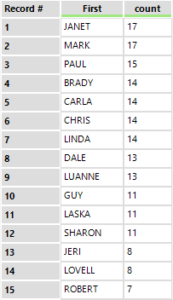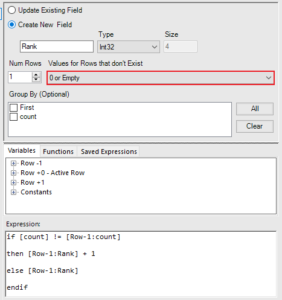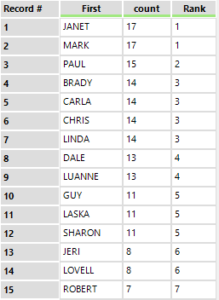## Using Multi-Row Formula to Rank Records in Alteryx

by Thomas Luthi

A challenge I encountered when coming to grips with Alteryx was to rank records based on a shared value.

For this exercise, we are trying to rank players according to their score. Simply sorting and ranking the records would give players with the same score different ranks – what if we want players with the same score to share the same rank?

Or in broader terms, how can I give all records that share a certain value the same rank?[count] refers to players’ scores.

Alteryx’ Multi-Row Formula calculates the value for one row based on values in rows above or below it. We want to create a new Field called Rank which starts at 1 and goes up by increments of 1 every time the value in the [Count]-column is different from that in the row above.

For that purpose we insert a Mulri-Row Formula Tool. Check “Create New Field” and name it appropriately – for our exercise, we name the field “Rank”. Then make sure you have selected “0 or empty” for Rows that don’t Exist. In the expression box, insert the following if-statement:

if [count] != [Row-1:count] then [Row-1:Rank] + 1 else [ROW-1:Rank] endifMulti-Row Formula

Because we selected “0 or Empty” for rows that don’t exist, Alteryx assumes there is a hypothetical row above the first whose value is 0. That’s what the formula will reference in the very first row, thus the first value in the new Rank column will be (0+1) = 1.Players Ranked according to ScoreThomas Luthi